## Graphs of Logarithmic Functions

### Learning Outcomes

• Determine the domain and range of a logarithmic function.
• Determine the x-intercept and vertical asymptote of a logarithmic function.
• Identify whether a logarithmic function is increasing or decreasing and give the interval.
• Identify the features of a logarithmic function that make it an inverse of an exponential function.
• Graph horizontal and vertical shifts of logarithmic functions.
• Graph stretches and compressions of logarithmic functions.
• Graph reflections of logarithmic functions.

Previously, we saw how creating a graphical representation of an exponential model gives us another layer of insight for predicting future events. How do logarithmic graphs give us insight into situations? Because every logarithmic function is the inverse function of an exponential function, we can think of every output on a logarithmic graph as the input for the corresponding inverse exponential equation. In other words, logarithms give the cause for an effect.

To illustrate, suppose we invest \$2500 in an account that offers an annual interest rate of 5% compounded continuously. We already know that the balance in our account for any year t can be found with the equation $A=2500{e}^{0.05t}$.

What if we wanted to know the year for any balance? We would need to create a corresponding new function by interchanging the input and the output; thus we would need to create a logarithmic model for this situation. By graphing the model, we can see the output (year) for any input (account balance). For instance, what if we wanted to know how many years it would take for our initial investment to double? The graph below shows this point.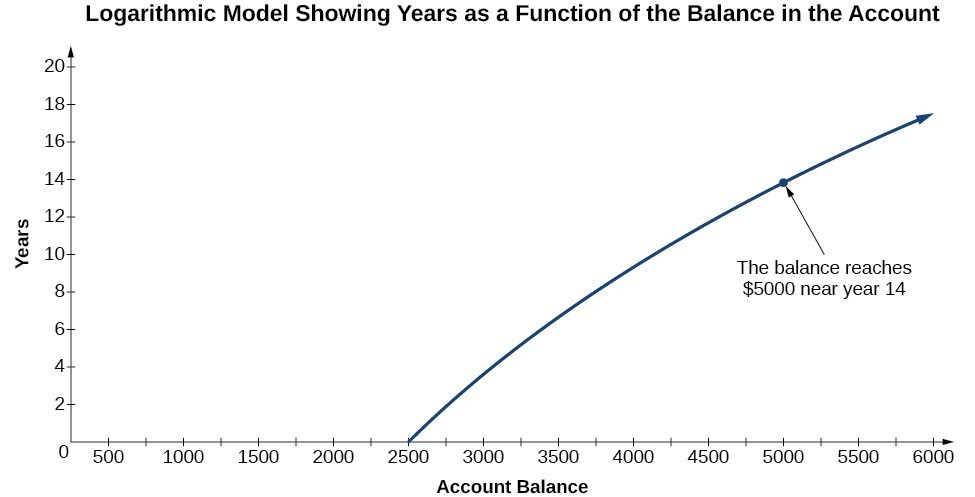In this section we will discuss the values for which a logarithmic function is defined and then turn our attention to graphing the family of logarithmic functions.

## Characteristics of Graphs of Logarithmic Functions

Before working with graphs, we will take a look at the domain (the set of input values) for which the logarithmic function is defined.

Recall that the exponential function is defined as $y={b}^{x}$ for any real number x and constant $b>0$, $b\ne 1$, where

• The domain of y is $\left(-\infty ,\infty \right)$.
• The range of y is $\left(0,\infty \right)$.

In the last section we learned that the logarithmic function $y={\mathrm{log}}_{b}\left(x\right)$ is the inverse of the exponential function $y={b}^{x}$. So, as inverse functions:

• The domain of $y={\mathrm{log}}_{b}\left(x\right)$ is the range of $y={b}^{x}$: $\left(0,\infty \right)$.
• The range of $y={\mathrm{log}}_{b}\left(x\right)$ is the domain of $y={b}^{x}$: $\left(-\infty ,\infty \right)$.

Transformations of the parent function $y={\mathrm{log}}_{b}\left(x\right)$ behave similarly to those of other functions. Just as with other parent functions, we can apply the four types of transformations—shifts, stretches, compressions, and reflections—to the parent function without loss of shape.

Previously we saw that certain transformations can change the range of $y={b}^{x}$. Similarly, applying transformations to the parent function $y={\mathrm{log}}_{b}\left(x\right)$ can change the domain. Therefore, when finding the domain of a logarithmic function, it is important to remember that the domain consists only of positive real numbers. That is, the value you are applying the logarithmic function to, also known as the argument of the logarithmic function, must be greater than zero.

For example, consider $f\left(x\right)={\mathrm{log}}_{4}\left(2x - 3\right)$. This function is defined for any values of x such that the argument, in this case $2x - 3$, is greater than zero. To find the domain, we set up an inequality and solve for x:

$\begin{array}{l}2x - 3>0\hfill & \text{Show the argument greater than zero}.\hfill \\ 2x>3\hfill & \text{Add 3}.\hfill \\ x>1.5\hfill & \text{Divide by 2}.\hfill \end{array}$

In interval notation, the domain of $f\left(x\right)={\mathrm{log}}_{4}\left(2x - 3\right)$ is $\left(1.5,\infty \right)$.

### How To: Given a logarithmic function, identify the domain

1. Set up an inequality showing the argument greater than zero.
2. Solve for x.
3. Write the domain in interval notation.

### Example: Identifying the Domain Resulting From a Logarithmic Shift

What is the domain of $f\left(x\right)={\mathrm{log}}_{2}\left(x+3\right)$?

### Try It

What is the domain of $f\left(x\right)={\mathrm{log}}_{5}\left(x - 2\right)+1$?

### Example: Identifying the Domain Resulting From a Logarithmic Shift and Reflection

What is the domain of $f\left(x\right)=\mathrm{log}\left(5 - 2x\right)$?

### Try It

What is the domain of $f\left(x\right)=\mathrm{log}\left(x - 5\right)+2$?

## Graphing a Logarithmic Function Using a Table of Values

Now that we have a feel for the set of values for which a logarithmic function is defined, we move on to graphing logarithmic functions. The family of logarithmic functions includes the parent function $y={\mathrm{log}}_{b}\left(x\right)$ along with all of its transformations: shifts, stretches, compressions, and reflections.

We begin with the parent function $y={\mathrm{log}}_{b}\left(x\right)$. Because every logarithmic function of this form is the inverse of an exponential function of the form $y={b}^{x}$, their graphs will be reflections of each other across the line $y=x$. To illustrate this, we can observe the relationship between the input and output values of $y={2}^{x}$ and its equivalent logarithmic form $x={\mathrm{log}}_{2}\left(y\right)$ in the table below.

 x –3 –2 –1 0 1 2 3 ${2}^{x}=y$ $\frac{1}{8}$ $\frac{1}{4}$ $\frac{1}{2}$ 1 2 4 8 ${\mathrm{log}}_{2}\left(y\right)=x$ –3 –2 –1 0 1 2 3

Using the inputs and outputs from the table above, we can build another table to observe the relationship between points on the graphs of the inverse functions $f\left(x\right)={2}^{x}$ and $g\left(x\right)={\mathrm{log}}_{2}\left(x\right)$.

 $f\left(x\right)={2}^{x}$ $\left(-3,\frac{1}{8}\right)$ $\left(-2,\frac{1}{4}\right)$ $\left(-1,\frac{1}{2}\right)$ $\left(0,1\right)$ $\left(1,2\right)$ $\left(2,4\right)$ $\left(3,8\right)$ $g\left(x\right)={\mathrm{log}}_{2}\left(x\right)$ $\left(\frac{1}{8},-3\right)$ $\left(\frac{1}{4},-2\right)$ $\left(\frac{1}{2},-1\right)$ $\left(1,0\right)$ $\left(2,1\right)$ $\left(4,2\right)$ $\left(8,3\right)$

As we would expect, the and y-coordinates are reversed for the inverse functions. The figure below shows the graphs of f and g.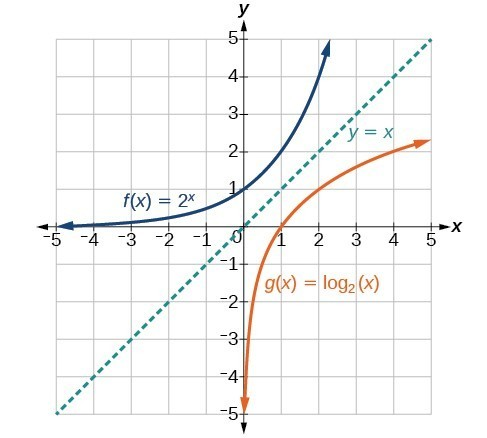Notice that the graphs of $f\left(x\right)={2}^{x}$ and $g\left(x\right)={\mathrm{log}}_{2}\left(x\right)$ are reflections about the line y = x since they are inverses of each other.

Observe the following from the graph:

• $f\left(x\right)={2}^{x}$ has a y-intercept at $\left(0,1\right)$ and $g\left(x\right)={\mathrm{log}}_{2}\left(x\right)$ has an x-intercept at $\left(1,0\right)$.
• The domain of $f\left(x\right)={2}^{x}$, $\left(-\infty ,\infty \right)$, is the same as the range of $g\left(x\right)={\mathrm{log}}_{2}\left(x\right)$.
• The range of $f\left(x\right)={2}^{x}$, $\left(0,\infty \right)$, is the same as the domain of $g\left(x\right)={\mathrm{log}}_{2}\left(x\right)$.

### A General Note: Characteristics of the Graph of the Parent Function $f\left(x\right)={\mathrm{log}}_{b}\left(x\right)$

For any real number x and constant > 0, $b\ne 1$, we can see the following characteristics in the graph of $f\left(x\right)={\mathrm{log}}_{b}\left(x\right)$:

• one-to-one function
• vertical asymptote: = 0
• domain: $\left(0,\infty \right)$
• range: $\left(-\infty ,\infty \right)$
• x-intercept: $\left(1,0\right)$ and key point $\left(b,1\right)$
• y-intercept: none
• increasing if $b>1$
• decreasing if 0 < < 1

The graphs below show how changing the base b in $f\left(x\right)={\mathrm{log}}_{b}\left(x\right)$ can affect the graphs. Observe that the graphs compress vertically as the value of the base increases. (Note: recall that the function $\mathrm{ln}\left(x\right)$ is base $e\approx \text{2}.\text{718}$. and $\mathrm{ln}\left(x\right)$ is base 10.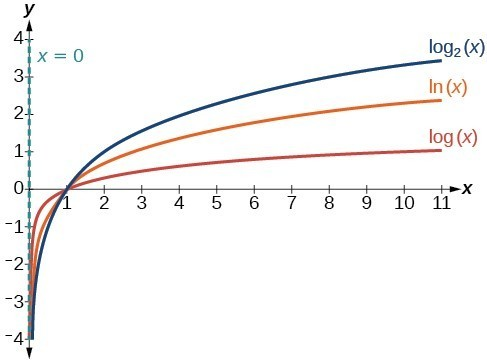The graphs of three logarithmic functions with different bases all greater than 1.

### How To: Given a logarithmic function Of the form $f\left(x\right)={\mathrm{log}}_{b}\left(x\right)$, graph the function

1. Draw and label the vertical asymptote, x = 0.
2. Plot the x-intercept, $\left(1,0\right)$.
3. Plot the key point $\left(b,1\right)$.
4. Draw a smooth curve through the points.
5. State the domain, $\left(0,\infty \right)$, the range, $\left(-\infty ,\infty \right)$, and the vertical asymptote, x = 0.

### Example: Graphing a Logarithmic Function Of the Form $f\left(x\right)={\mathrm{log}}_{b}\left(x\right)$

Graph $f\left(x\right)={\mathrm{log}}_{5}\left(x\right)$. State the domain, range, and asymptote.

### Try It

Graph $f\left(x\right)={\mathrm{log}}_{\frac{1}{5}}\left(x\right)$. State the domain, range, and asymptote.

## Horizontal and Vertical Shifts of Logarithmic Functions

As we mentioned in the beginning of the section, transformations of logarithmic functions behave similar to those of other parent functions. We can shift, stretch, compress, and reflect the parent function $y={\mathrm{log}}_{b}\left(x\right)$ without loss of shape.

### Graphing a Horizontal Shift of $f\left(x\right)={\mathrm{log}}_{b}\left(x\right)$

When a constant c is added to the input of the parent function $f\left(x\right)=\text{log}_{b}\left(x\right)$, the result is a horizontal shift c units in the opposite direction of the sign on c. To visualize horizontal shifts, we can observe the general graph of the parent function $f\left(x\right)={\mathrm{log}}_{b}\left(x\right)$ alongside the shift left, $g\left(x\right)={\mathrm{log}}_{b}\left(x+c\right)$, and the shift right, $h\left(x\right)={\mathrm{log}}_{b}\left(x-c\right)$ where > 0.The graphs below summarize the changes in the x-intercepts, vertical asymptotes, and equations of a logarithmic function that has been shifted either right or left.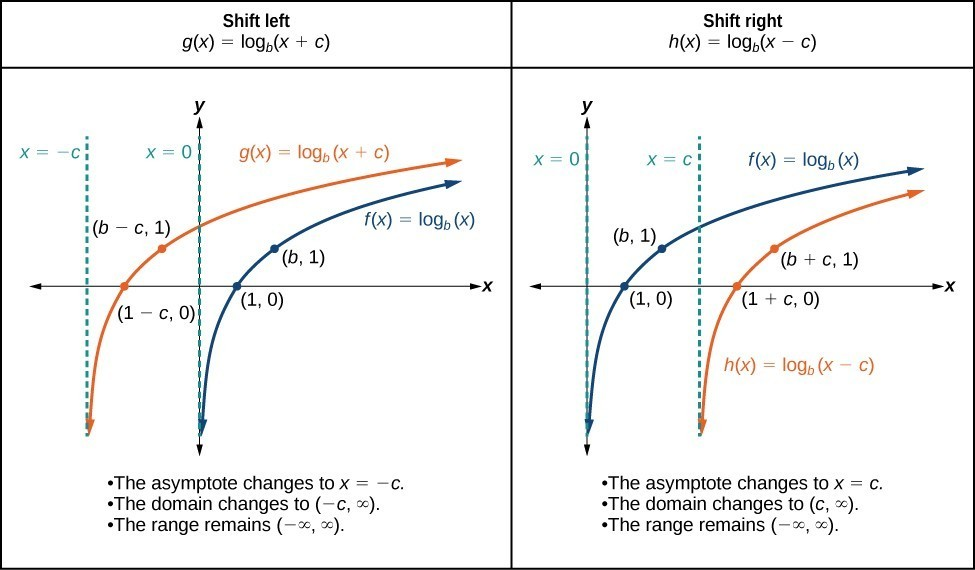### A General Note: Horizontal Shifts of the Parent Function $y=\text{log}_{b}\left(x\right)$

For any constant c, the function $f\left(x\right)={\mathrm{log}}_{b}\left(x+c\right)$

• shifts the parent function $y={\mathrm{log}}_{b}\left(x\right)$ left c units if > 0.
• shifts the parent function $y={\mathrm{log}}_{b}\left(x\right)$ right c units if < 0.
• has the vertical asymptote = –c.
• has domain $\left(-c,\infty \right)$.
• has range $\left(-\infty ,\infty \right)$.

### How To: Given a logarithmic function Of the form $f\left(x\right)={\mathrm{log}}_{b}\left(x+c\right)$, graph the Horizontal Shift

1. Identify the horizontal shift:
• If c > 0, shift the graph of $f\left(x\right)={\mathrm{log}}_{b}\left(x\right)$ left c units.
• If < 0, shift the graph of $f\left(x\right)={\mathrm{log}}_{b}\left(x\right)$ right c units.
2. Draw the vertical asymptote = –c.
3. Identify three key points from the parent function. Find new coordinates for the shifted functions by subtracting c from the x coordinate in each point.
4. Label the three points.
5. The domain is $\left(-c,\infty \right)$, the range is $\left(-\infty ,\infty \right)$, and the vertical asymptote is = –c.

### Example: Graphing a Horizontal Shift of the Parent Function $y=\text{log}_{b}\left(x\right)$

Sketch the horizontal shift $f\left(x\right)={\mathrm{log}}_{3}\left(x - 2\right)$ alongside its parent function. Include the key points and asymptotes on the graph. State the domain, range, and asymptote.

### Try It

Sketch a graph of $f\left(x\right)={\mathrm{log}}_{3}\left(x+4\right)$ alongside its parent function. Include the key points and asymptotes on the graph. State the domain, range, and asymptote.

### Graphing a Vertical Shift of $y=\text{log}_{b}\left(x\right)$

When a constant d is added to the parent function $f\left(x\right)={\mathrm{log}}_{b}\left(x\right)$, the result is a vertical shift d units in the direction of the sign of d. To visualize vertical shifts, we can observe the general graph of the parent function $f\left(x\right)={\mathrm{log}}_{b}\left(x\right)$ alongside the shift up, $g\left(x\right)={\mathrm{log}}_{b}\left(x\right)+d$, and the shift down, $h\left(x\right)={\mathrm{log}}_{b}\left(x\right)-d$.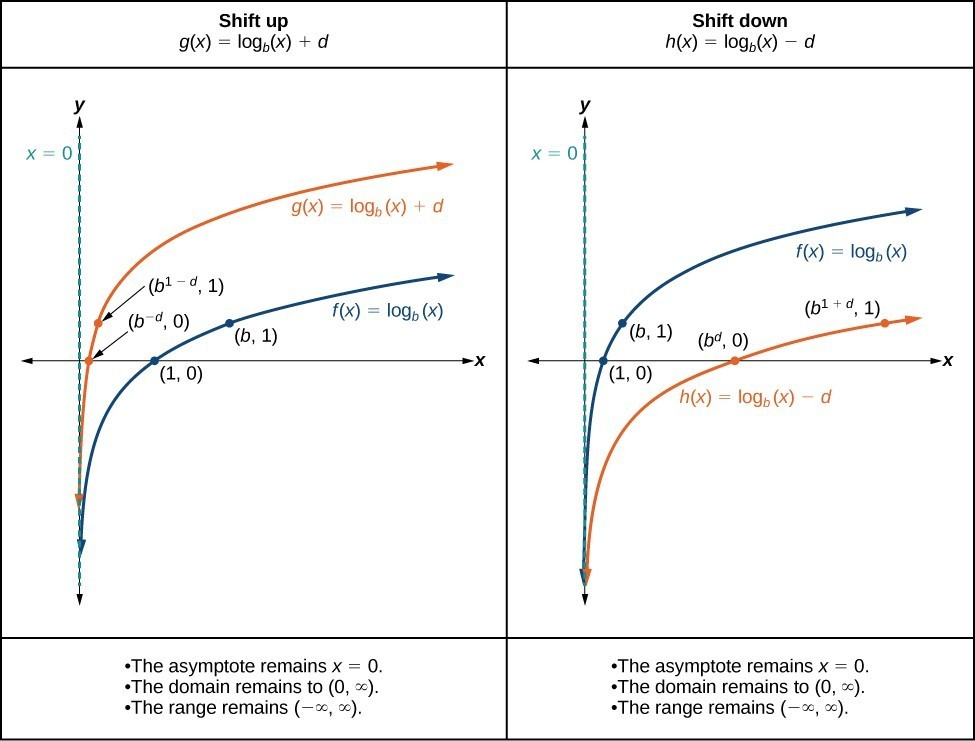### A General Note: Vertical Shifts of the Parent Function $y=\text{log}_{b}\left(x\right)$

For any constant d, the function $f\left(x\right)={\mathrm{log}}_{b}\left(x\right)+d$

• shifts the parent function $y={\mathrm{log}}_{b}\left(x\right)$ up d units if > 0.
• shifts the parent function $y={\mathrm{log}}_{b}\left(x\right)$ down d units if < 0.
• has the vertical asymptote = 0.
• has domain $\left(0,\infty \right)$.
• has range $\left(-\infty ,\infty \right)$.

### How To: Given a logarithmic function Of the form $f\left(x\right)={\mathrm{log}}_{b}\left(x\right)+d$, graph the Vertical Shift

1. Identify the vertical shift:
• If > 0, shift the graph of $f\left(x\right)={\mathrm{log}}_{b}\left(x\right)$ up d units.
• If < 0, shift the graph of $f\left(x\right)={\mathrm{log}}_{b}\left(x\right)$ down units.
2. Draw the vertical asymptote = 0.
3. Identify three key points from the parent function. Find new coordinates for the shifted functions by adding d to the coordinate of each point.
4. Label the three points.
5. The domain is $\left(0,\infty \right)$, the range is $\left(-\infty ,\infty \right)$, and the vertical asymptote is = 0.

### Example: Graphing a Vertical Shift of the Parent Function $y=\text{log}_{b}\left(x\right)$

Sketch a graph of $f\left(x\right)={\mathrm{log}}_{3}\left(x\right)-2$ alongside its parent function. Include the key points and asymptote on the graph. State the domain, range, and asymptote.

### Try It

Sketch a graph of $f\left(x\right)={\mathrm{log}}_{2}\left(x\right)+2$ alongside its parent function. Include the key points and asymptote on the graph. State the domain, range, and asymptote.

## Stretching, Compressing, or Reflecting a Logarithmic Function

### Graphing Stretches and Compressions of $y=\text{log}_{b}\left(x\right)$

When the parent function $f\left(x\right)={\mathrm{log}}_{b}\left(x\right)$ is multiplied by a constant a > 0, the result is a vertical stretch or compression of the original graph. To visualize stretches and compressions, we set > 1 and observe the general graph of the parent function $f\left(x\right)={\mathrm{log}}_{b}\left(x\right)$ alongside the vertical stretch, $g\left(x\right)=a{\mathrm{log}}_{b}\left(x\right)$, and the vertical compression, $h\left(x\right)=\frac{1}{a}{\mathrm{log}}_{b}\left(x\right)$.The graphs below summarize the key features of the resulting graphs of vertical stretches and compressions of logarithmic functions.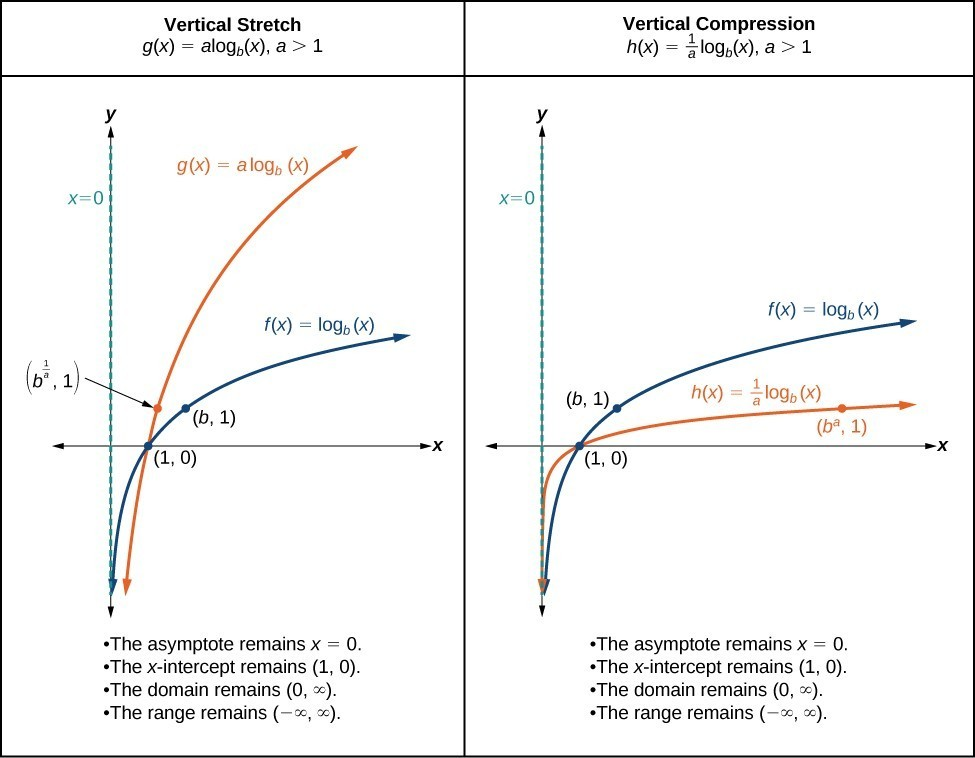### A General Note: Vertical Stretches and Compressions of the Parent Function $y=\text{log}_{b}\left(x\right)$

For any constant a > 1, the function $f\left(x\right)=a{\mathrm{log}}_{b}\left(x\right)$

• stretches the parent function $y={\mathrm{log}}_{b}\left(x\right)$ vertically by a factor of a if > 1.
• compresses the parent function $y={\mathrm{log}}_{b}\left(x\right)$ vertically by a factor of a if 0 < < 1.
• has the vertical asymptote = 0.
• has the x-intercept $\left(1,0\right)$.
• has domain $\left(0,\infty \right)$.
• has range $\left(-\infty ,\infty \right)$.

### How To: Given a logarithmic function Of the form $f\left(x\right)=a{\mathrm{log}}_{b}\left(x\right)$, $a>0$, graph the Stretch or Compression

1. Identify the vertical stretch or compression:
• If $|a|>1$, the graph of $f\left(x\right)={\mathrm{log}}_{b}\left(x\right)$ is stretched by a factor of a units.
• If $|a|<1$, the graph of $f\left(x\right)={\mathrm{log}}_{b}\left(x\right)$ is compressed by a factor of a units.
2. Draw the vertical asymptote = 0.
3. Identify three key points from the parent function. Find new coordinates for the shifted functions by multiplying the y coordinates in each point by a.
4. Label the three points.
5. The domain is $\left(0,\infty \right)$, the range is $\left(-\infty ,\infty \right)$, and the vertical asymptote is x = 0.

### Example: Graphing a Stretch or Compression of the Parent Function $y=\text{log}_{b}\left(x\right)$

Sketch the graph of $f\left(x\right)=2{\mathrm{log}}_{4}\left(x\right)$ alongside its parent function. Include the key points and asymptote on the graph. State the domain, range, and asymptote.

### Try It

Sketch a graph of $f\left(x\right)=\frac{1}{2}{\mathrm{log}}_{4}\left(x\right)$ alongside its parent function. Include the key points and asymptote on the graph. State the domain, range, and asymptote.

### Example: Combining a Shift and a Stretch

Sketch the graph of $f\left(x\right)=5\mathrm{log}\left(x+2\right)$. State the domain, range, and asymptote.

### Try It

Sketch a graph of the function $f\left(x\right)=3\mathrm{log}\left(x - 2\right)+1$. State the domain, range, and asymptote.

### Graphing Reflections of $f\left(x\right)={\mathrm{log}}_{b}\left(x\right)$

When the parent function $f\left(x\right)={\mathrm{log}}_{b}\left(x\right)$ is multiplied by –1, the result is a reflection about the x-axis. When the input is multiplied by –1, the result is a reflection about the y-axis. To visualize reflections, we restrict > 1 and observe the general graph of the parent function $f\left(x\right)={\mathrm{log}}_{b}\left(x\right)$ alongside the reflection about the x-axis, $g\left(x\right)={\mathrm{-log}}_{b}\left(x\right)$, and the reflection about the y-axis, $h\left(x\right)={\mathrm{log}}_{b}\left(-x\right)$.

The graphs below summarize the key characteristics of reflecting $f(x) = \log_{b}{x}$ horizontally and vertically.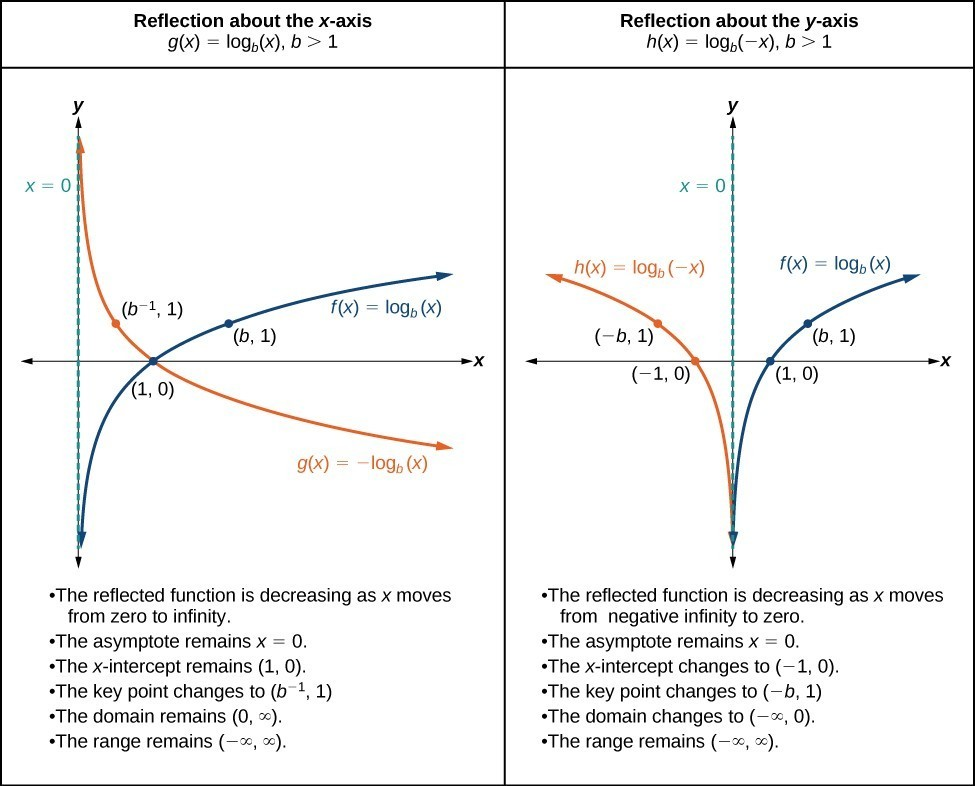### A General Note: Reflections of the Parent Function $y=\text{log}_{b}\left(x\right)$

The function $f\left(x\right)={\mathrm{-log}}_{b}\left(x\right)$

• reflects the parent function $y={\mathrm{log}}_{b}\left(x\right)$ about the x-axis.
• has domain $\left(0,\infty \right)$, range, $\left(-\infty ,\infty \right)$, and vertical asymptote = 0 which are unchanged from the parent function.

The function $f\left(x\right)={\mathrm{log}}_{b}\left(-x\right)$

• reflects the parent function $y={\mathrm{log}}_{b}\left(x\right)$ about the y-axis.
• has domain $\left(-\infty ,0\right)$.
• has range $\left(-\infty ,\infty \right)$ and vertical asymptote = 0 which are unchanged from the parent function.

### How To: Given a logarithmic function with the parent function $f\left(x\right)={\mathrm{log}}_{b}\left(x\right)$, graph a Reflection

$\text{If }f\left(x\right)=-{\mathrm{log}}_{b}\left(x\right)$ $\text{If }f\left(x\right)={\mathrm{log}}_{b}\left(-x\right)$
1. Draw the vertical asymptote, = 0. 1. Draw the vertical asymptote, = 0.
2. Plot the x-intercept, $\left(1,0\right)$. 2. Plot the x-intercept, $\left(1,0\right)$.
3. Reflect the graph of the parent function $f\left(x\right)={\mathrm{log}}_{b}\left(x\right)$ about the x-axis. 3. Reflect the graph of the parent function $f\left(x\right)={\mathrm{log}}_{b}\left(x\right)$ about the y-axis.
4. Draw a smooth curve through the points. 4. Draw a smooth curve through the points.
5. State the domain $\left(0,\infty \right)$, the range $\left(-\infty ,\infty \right)$, and the vertical asymptote = 0. 5. State the domain $\left(-\infty ,0\right)$, the range $\left(-\infty ,\infty \right)$, and the vertical asymptote = 0.

### Example: Graphing a Reflection of a Logarithmic Function

Sketch a graph of $f\left(x\right)=\mathrm{log}\left(-x\right)$ alongside its parent function. Include the key points and asymptote on the graph. State the domain, range, and asymptote.

### Try It

Graph $f\left(x\right)=-\mathrm{log}\left(-x\right)$. State the domain, range, and asymptote.

### How To: Given a logarithmic equation, use a graphing calculator to approximate solutions

1. Press [Y=]. Enter the given logarithmic equation or equations as Y1= and, if needed, Y2=.
2. Press [GRAPH] to observe the graphs of the curves and use [WINDOW] to find an appropriate view of the graphs, including their point(s) of intersection.
3. To find the value of x, we compute the point of intersection. Press [2ND] then [CALC]. Select “intersect” and press [ENTER] three times. The point of intersection gives the value of for the point(s) of intersection.

### Example: Approximating the Solution of a Logarithmic Equation

Solve $4\mathrm{ln}\left(x\right)+1=-2\mathrm{ln}\left(x - 1\right)$ graphically. Round to the nearest thousandth.

### Try It

Solve $5\mathrm{log}\left(x+2\right)=4-\mathrm{log}\left(x\right)$ graphically. Round to the nearest thousandth.

## Summarizing Transformations of Logarithmic Functions

Now that we have worked with each type of transformation for the logarithmic function, we can summarize each in the table below to arrive at the general equation for transforming exponential functions.

Transformations of the Parent Function $y={\mathrm{log}}_{b}\left(x\right)$
Transformation Form
Shift

• Horizontally c units to the left
• Vertically d units up
$y={\mathrm{log}}_{b}\left(x+c\right)+d$
Stretch and Compression

• Stretch if $|a|>1$
• Compression if $|a|<1$
$y=a{\mathrm{log}}_{b}\left(x\right)$
Reflection about the x-axis $y=-{\mathrm{log}}_{b}\left(x\right)$
Reflection about the y-axis $y={\mathrm{log}}_{b}\left(-x\right)$
General equation for all transformations $y=a{\mathrm{log}}_{b}\left(x+c\right)+d$

### A General Note: Transformations of Logarithmic Functions

All transformations of the parent logarithmic function $y={\mathrm{log}}_{b}\left(x\right)$ have the form

$f\left(x\right)=a{\mathrm{log}}_{b}\left(x+c\right)+d$

where the parent function, $y={\mathrm{log}}_{b}\left(x\right),b>1$, is

• shifted vertically up d units.
• shifted horizontally to the left c units.
• stretched vertically by a factor of |a| if |a| > 0.
• compressed vertically by a factor of |a| if 0 < |a| < 1.
• reflected about the x-axis when < 0.

For $f\left(x\right)=\mathrm{log}\left(-x\right)$, the graph of the parent function is reflected about the y-axis.

### Example: Finding the Vertical Asymptote of A LogarithmIC Function

What is the vertical asymptote of $f\left(x\right)=-2{\mathrm{log}}_{3}\left(x+4\right)+5$?

### Try It

What is the vertical asymptote of $f\left(x\right)=3+\mathrm{ln}\left(x - 1\right)$?

### Example: Finding the Equation from a Graph

Find a possible equation for the common logarithmic function graphed below.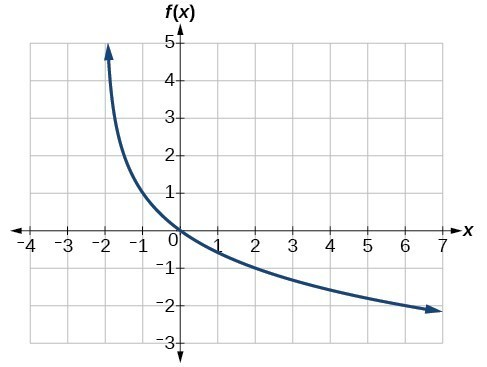### Try It

Give the equation of the logarithmic function graphed below.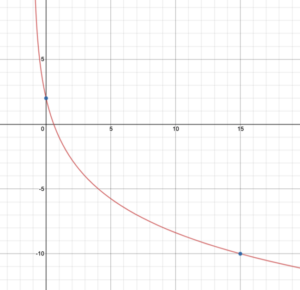### Try It

Give the equation of the natural logarithm graphed below.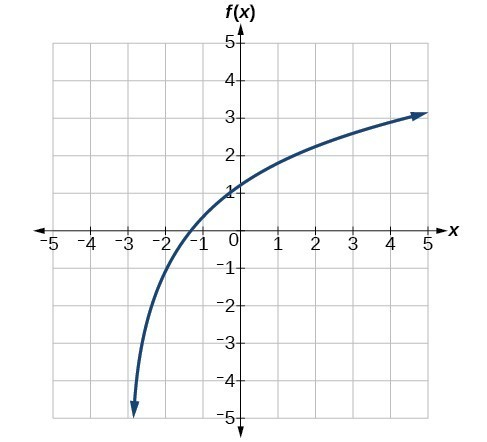### Q & A

Is it possible to tell the domain and range and describe the end behavior of a function just by looking at the graph?

Yes if we know the function is a general logarithmic function. For example, look at the graph in the previous example. The graph approaches x = –3 (or thereabouts) more and more closely, so x = –3 is, or is very close to, the vertical asymptote. It approaches from the right, so the domain is all points to the right, $\left\{x|x>-3\right\}$. The range, as with all general logarithmic functions, is all real numbers. And we can see the end behavior because the graph goes down as it goes left and up as it goes right. The end behavior is as $x\to -{3}^{+},f\left(x\right)\to -\infty$ and as $x\to \infty ,f\left(x\right)\to \infty$.

## Key Equations

 General Form for the Transformation of the Parent Logarithmic Function $\text{ }f\left(x\right)={\mathrm{log}}_{b}\left(x\right)$ $f\left(x\right)=a{\mathrm{log}}_{b}\left(x+c\right)+d$

## Key Concepts

• To find the domain of a logarithmic function, set up an inequality showing the argument greater than zero and solve for x.
• The graph of the parent function $f\left(x\right)={\mathrm{log}}_{b}\left(x\right)$ has an x-intercept at $\left(1,0\right)$, domain $\left(0,\infty \right)$, range $\left(-\infty ,\infty \right)$, vertical asymptote = 0, and
• if > 1, the function is increasing.
• if 0 < < 1, the function is decreasing.
• The equation $f\left(x\right)={\mathrm{log}}_{b}\left(x+c\right)$ shifts the parent function $y={\mathrm{log}}_{b}\left(x\right)$ horizontally
• left c units if > 0.
• right c units if < 0.
• The equation $f\left(x\right)={\mathrm{log}}_{b}\left(x\right)+d$ shifts the parent function $y={\mathrm{log}}_{b}\left(x\right)$ vertically
• up d units if > 0.
• down d units if < 0.
• For any constant > 0, the equation $f\left(x\right)=a{\mathrm{log}}_{b}\left(x\right)$
• stretches the parent function $y={\mathrm{log}}_{b}\left(x\right)$ vertically by a factor of a if |a| > 1.
• compresses the parent function $y={\mathrm{log}}_{b}\left(x\right)$ vertically by a factor of a if |a| < 1.
• When the parent function $y={\mathrm{log}}_{b}\left(x\right)$ is multiplied by –1, the result is a reflection about the x-axis. When the input is multiplied by –1, the result is a reflection about the y-axis.
• The equation $f\left(x\right)=-{\mathrm{log}}_{b}\left(x\right)$ represents a reflection of the parent function about the x-axis.
• The equation $f\left(x\right)={\mathrm{log}}_{b}\left(-x\right)$ represents a reflection of the parent function about the y-axis.
• A graphing calculator may be used to approximate solutions to some logarithmic equations.
• All transformations of the logarithmic function can be summarized by the general equation $f\left(x\right)=a{\mathrm{log}}_{b}\left(x+c\right)+d$.
• Given an equation with the general form $f\left(x\right)=a{\mathrm{log}}_{b}\left(x+c\right)+d$, we can identify the vertical asymptote = –c for the transformation.
• Using the general equation $f\left(x\right)=a{\mathrm{log}}_{b}\left(x+c\right)+d$, we can write the equation of a logarithmic function given its graph.